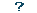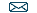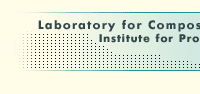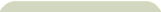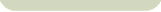Related Communities:Constructing Hypothesis Lattices for Virtual Experiments in Data Intensive Research

#### Constructing Hypothesis Lattices for Virtual Experiments in Data Intensive Research

 Author(s): Dmitry Kovalev, Sergey Stupnikov Published: Proc. 2019 Ivannikov Memorial Workshop (IVMEM), P. 14 – 17. IEEE, 2019 Abstract: Data intensive research is increasingly dependent on the explicit use of hypotheses, simulations and computational models. This paper is devoted to the development of infrastructure for explicit management of virtual experiments and research hypotheses. In particular, hypothesis lattices construction issues are considered. Basic concepts for working with research hypotheses such as hypotheses structure, its basic properties, causal correspondence of equations and variables over the defined structures are provided. The notion of hypotheses lattice is presented as a graph whose vertices are hypotheses, edges are the derived by relationship between hypotheses. An algorithm for constructing hypothesis lattices in virtual experiments is presented. A proof of the proposition on the complexity of the algorithm for constructing a lattice of hypotheses is provided. The developed method for constructing hypothesis lattices is implemented as a program component in the Python3 language. Download: [ https://ieeexplore.ieee.org/document/8880738 ]Supported by Synthesis Group